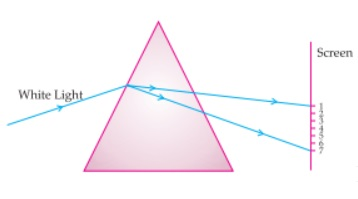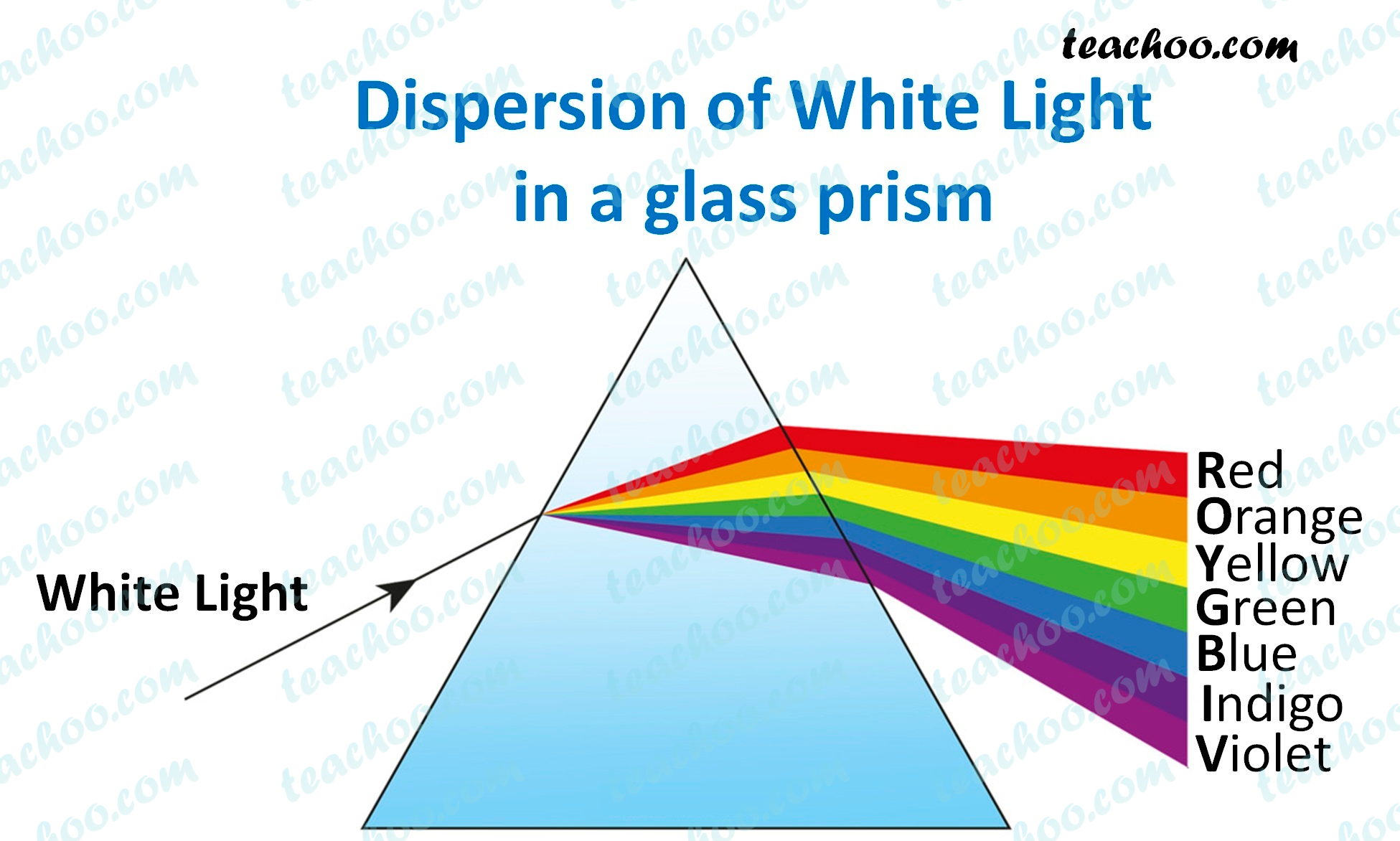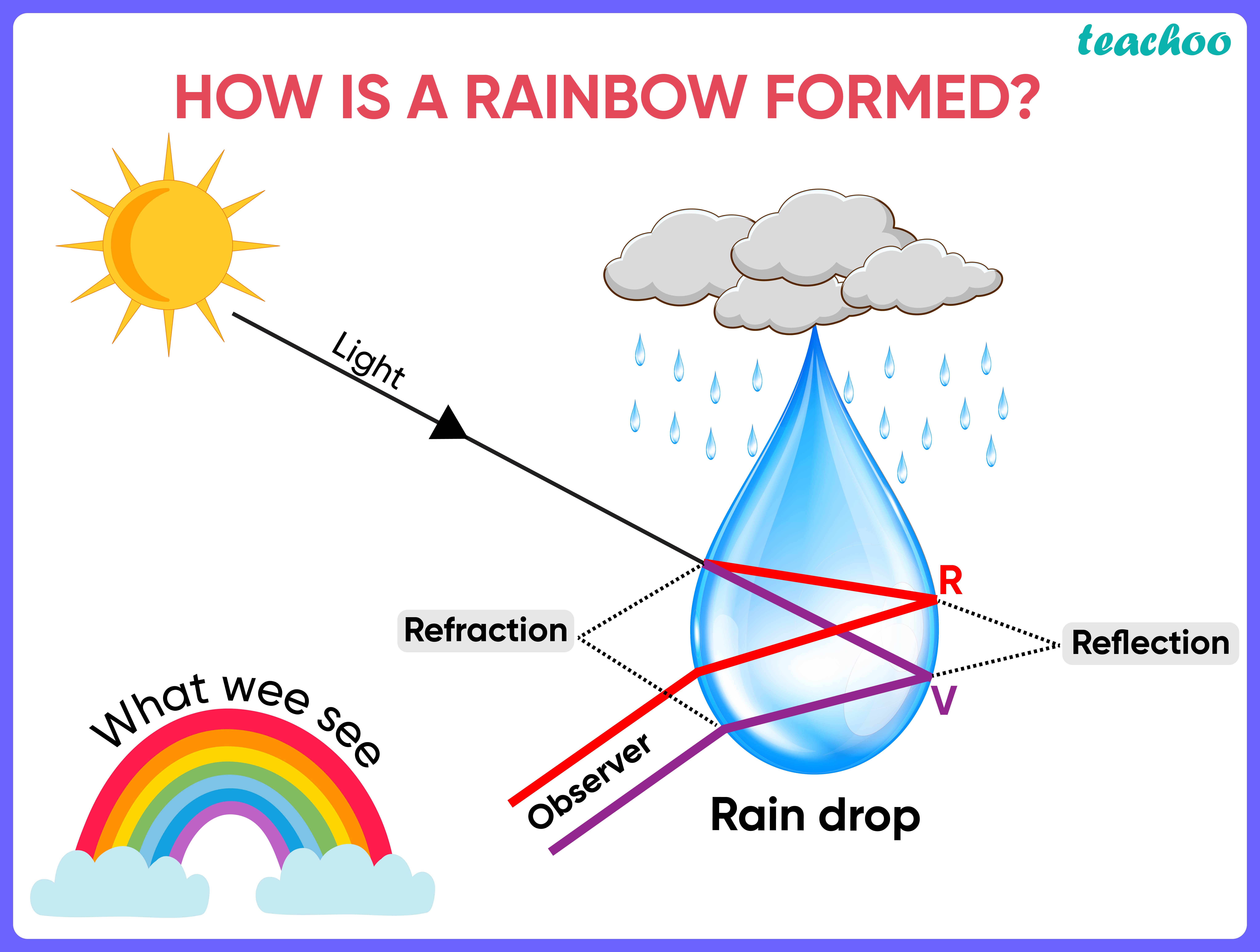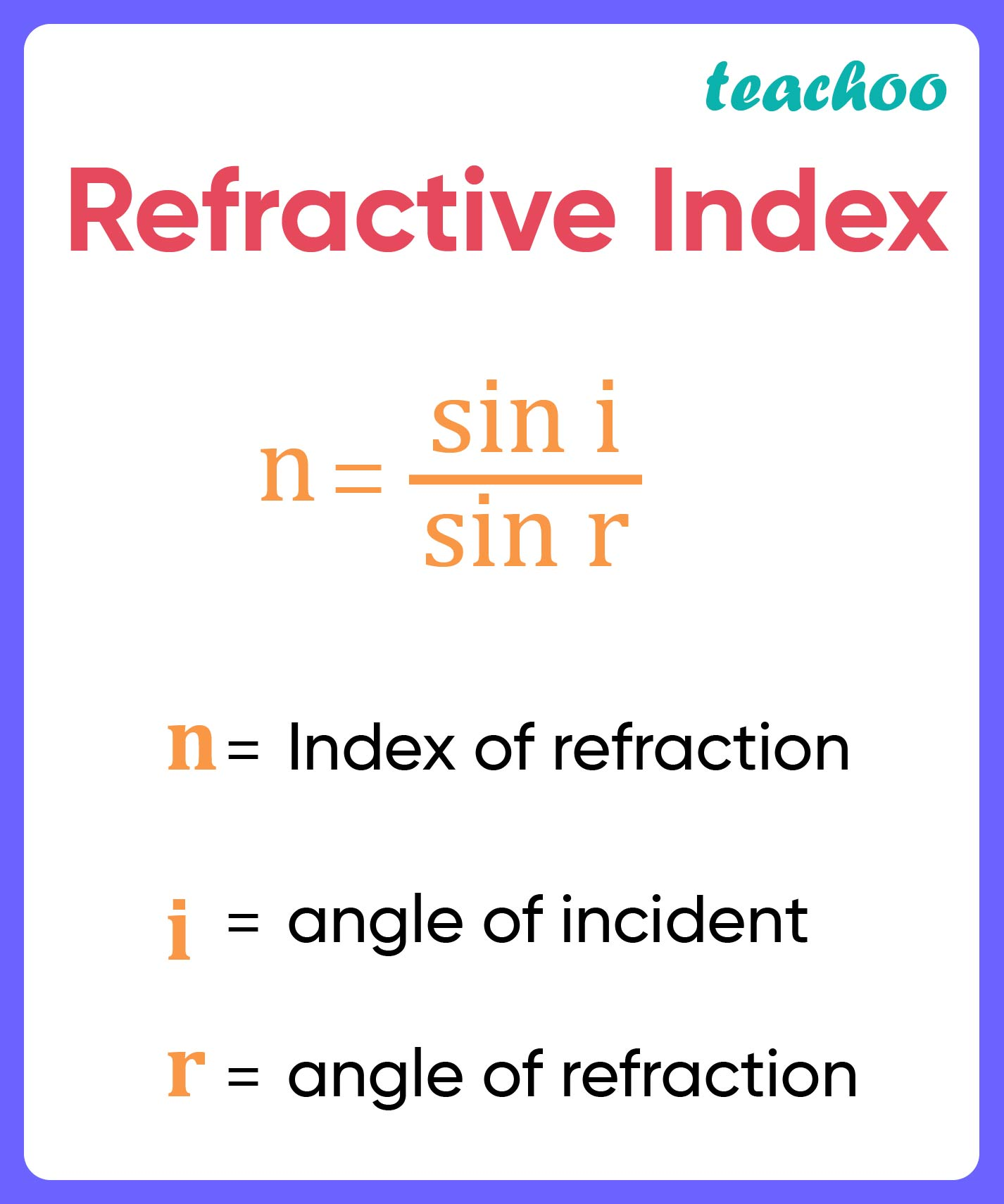Case Based Questions (MCQ)

Class 10
Chapter 11 Class 10 - Human Eye and Colourful World

##(adsbygoogle = window.adsbygoogle || []).push({});

### (iv) refraction of lightIn a prism, white light disperses into 7 different colors.

So, the correct answer is (ii)

### (iii) Blue colour of skyLet’s look at each of the options

• (i) Formation of rainbow - Rainbow is caused by dispersion of sunlight by tiny water droplets present in the atmosphere .
Since it is caused by dispersion, this option is correct

• (ii) Twinkling of stars   - Stars twinkle due to atmospheric refraction.
Since it is not caused by dispersion, this option is incorrect

• (iii) Blue colour of sky - Color of sky is blue due to scattering of light
Since it is not caused by dispersion, this option is incorrect

• (iv) Advance sunrise   - This is due to atmospheric refraction

So, the correct answer is (i)

### (iv) All the colours of the white light move with different speed in vacuum.

Let’s look at all the options one by one

• (i) White light consists of seven colours - This is true . White light disperses into 7 colors when passing throw a prism.• (ii) Violet colour suffers minimum deviation - This is false . Red light suffers least deviation, violet light suffers max deviation.

• (iii) Red light suffers maximum deviation - This is false . Red light suffers least deviation, violet light suffers max deviation.

• (iv) All the colours of the white light move with different speed in vacuum - All colors of light moves at the same speed in vaccum. So, this is also false.

So, the correct answer is (i)

### (iv) All the colors have the same wavelength.

Ans.Dispersion is caused because

• White light is composed of 7 colours . In vacuum, these 7 colours travel with the same speed (c=3 x 10 8 ).
• In any medium, due to refractive index, speed of light changes (Since n=c/c’).
• For white light, all 7 colors have different wavelengths , thus different refractive index, thus different speed of light .

Hence, we can say that all colors do not travel with the same speed of light.

So, the correct answer is (iii)

## (iv) All of the above

Ans.

Let’s look at all the options one by one

(I) The prism behaves the same as that of a rectangular  glass slab

• A prism is made up of glass in which  the faces are at a certain angle but in the case of  rectangular glass slabs , the opposite faces are parallel  to each other.
• As the light enters in the prism, all  the colours travel with the different speed because glass is a denser medium as compared to  air
• Dispersion of light is not observed in case of a rectangular glass slab

So, both the prism and rectangular glass slab behave differently. Thus, this statement is incorrect.

(II) All the colours have different angles of deviation in case of dispersion through prism

• Angles of deviation depends on the frequency of light. Since all colors have different frequencies, thus, all colours have different angles of  deviation  .

Thus, this statement is correct

(III) All the colours travel with the same speed of  light in glass

• Speed of light depends on the frequency of light. Since all colors have different frequencies, thus, all colours have different speeds.

Thus, this statement is incorrect.

(IV) Dispersion of light is observed in the case of  rectangular glass slabs

• Dispersion is not observed in a rectangular glass slab, rather later displacement is observed.

Thus, this statement is incorrect.

Since only statement (II) is correct.

So, the correct answer is (i)

Introducing your new favourite teacher - Teachoo Black, at only ₹83 per month